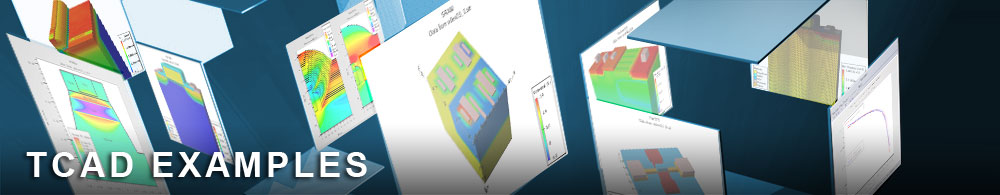Large Signal Output Power Analysis

ganfetex11.in : Large Signal Output Power Analysis

Requires: Blaze
Minimum Versions: Atlas 5.28.1.R

This example describes how to simulate typical 10 GHz large signal measured performance quantities related to power output, such as power out versus power in, power gain and amplifier efficiency as the device is over-driven into high distortion. The example also plots the output signal shape versus time for each of the ten input signal levels simulated.

Ten large signal input amplitudes are defined using WAVEFORM statements. Each of these waveforms are applied to the gate in order of increasing amplitude by using the LOOP statements. After each waveform simulation is complete, a number of large signal parameters are calculated using a number of EXTRACT statments.

First the peak input and output voltages are extracted, followed by the exact times at which these peak voltages occurred. Then the input and output currents are extracted at the same times as the peak voltages occur. It is important to extract the currents at the same time as the peak voltages in order to take account of the phase between current and voltage, so that the correct power values can be calculated. For example, if the current is zero at the time the peak output voltage occurs, the calculated power is correctly calculated to be also zero, since in this case the output signal would be purely reactive.

After each waveform simulation, all the calculated quantities are entered into a data file format using SYSTEM statements. At the end of the last simulation, the tonyplot format data file is complete and the results are displayed as a number of plots.

To load and run this example, select the Load button in DeckBuild > Examples. This will copy the input file and any support files to your current working directory. Select the Run button in DeckBuild to execute the example.